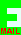## DIAGONALS

I have a rectangular block of wood (that is, each of the six faces are rectangles). The diagonals across the faces measure exactly 40, 43 and 51 mm. What is the length of the diagonal across the centre of the block?

Click this symbolfor my email address.
Closing date: 5th December 2007.

```If A,B,C are the squares of the three sides, the answer is the
square-root of A+B+C.  We don't need to work out the sides, which
are irrational numbers.

Pythagoras tells us that:

A+B   = 40 x 40 = 1600
B+C = 43 x 43 = 1849
A  +C = 51 x 51 = 2601